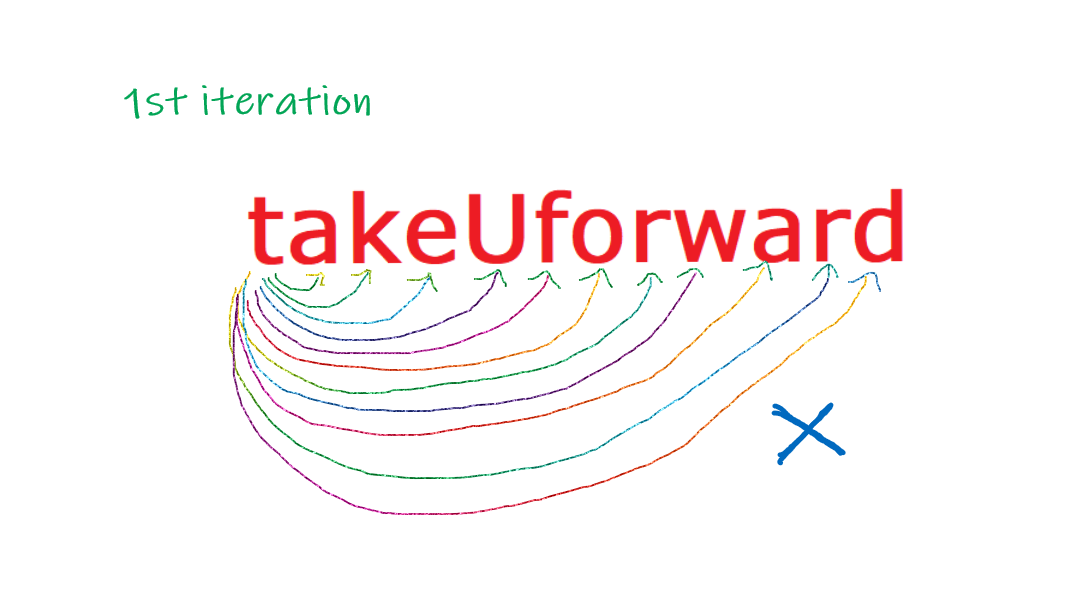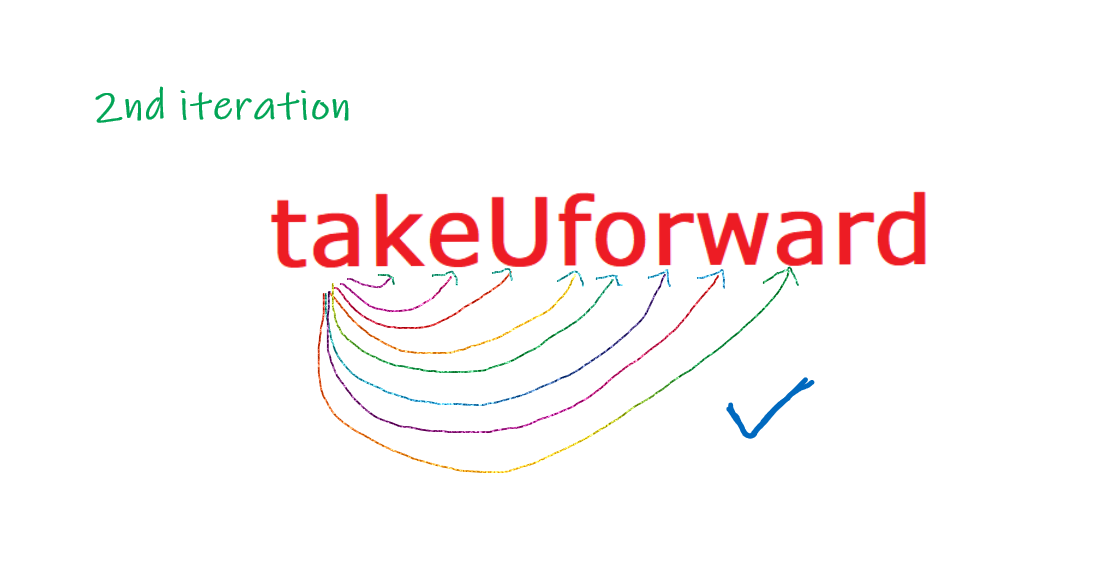# Leftmost Repeating Character in a String

Problem Statement: Given a string, find the leftmost repeating character of the string. i.e The first character that appeared again in the further string.With the reference to the above image, we can see the character ‘a’ is the left-most repeating element and the character ‘r’ is the second left-most repeating character.

Examples:

```Example 1:
Input: takeUforward
Output: a
Explanation: Character ‘a’ is the left-most repeating character or the first repeating character.

Example 2:

Input: programming
Output: r
Explanation: Character ‘r’ is the left-most repeating character or the first repeating character.
```

### Solution

Disclaimer: Don’t jump directly to the solution, try it out yourself first.

### Solution 1: Naive approach

Approach

• Select the first character of the string and compare it with every other character in            the string.
• If the current character matches with any of the further characters present in the string then immediately return / Print that character.
• Else, if all the characters are traversed or the string is empty then return or print -1.
• Return the answer.In the 1st iteration, there is no matching character in the entire string so we did not find a match for the 1st character of the string.

Now, with the 2nd character of the string, the 2nd iteration.Code:

## C++ Code

``````#include <bits/stdc++.h>

using namespace std;
int main() {
string str = "takeUforward";
int flag = 0;
for (int i = 0; i < str.size(); i++) {
for (int j = i + 1; j < str.size(); j++) {
if (str[i] == str[j]) {
cout << "The left-most repeating character is :" << " " << str[i];
flag = 1;
break;
}
}
if (flag == 1) {
break;
}
}
return 0;
}
``````

Output: The left-most repeating character is : a

Time Complexity: O(N^2)

Space Complexity: O(1)

## Java Code

``````import java.util.*;
class TUF {
public static void main(String[] args) {
String str = "takeUforward";
int flag = 0;
for (int i = 0; i < str.length(); i++) {
for (int j = i + 1; j < str.length(); j++) {
if (str.charAt(i) == str.charAt(j)) {
System.out.print("The left-most repeating character is :" + " " +
str.charAt(i));
flag = 1;
break;
}
}
if (flag == 1) {
break;
}
}
}
}
``````

Output: The left-most repeating character is : a

Time Complexity: O(N^2)

Space Complexity: O(1)

### Solution 2: Using Frequency Array

Approach

• We will create a frequency array of size 256 i.e (All symbols and characters’ ASCII values)
• We will traverse the string from left to right.
• For every encountered character we will increment the count of the frequency of that character in our frequency array.
• Once the entire string is traversed, We will Strat another loop of size 256 to check the frequency array and if the value of any index is greater than 1, We will return the index and after typecasting that index into the character we will get our answer.

Code:

## C++ Code

``````#include <bits/stdc++.h>

using namespace std;
#define NO_OF_CHARS 256

int firstRepeating(string & str) {
int firstIndex[NO_OF_CHARS] = {0};

for (int i = 0; i < str.size(); i++) {
firstIndex[str[i]]++;
}
for (int i = 0; i < str.size(); i++) {
if (firstIndex[str[i]] > 1) {
return i;
}
}
return -1;
}

int main() {
string str = "takeuforward";
int index = (firstRepeating(str));

if (index == -1)
cout << "Either all characters are distinct or string is empty";
else
cout << "The left-most repeating character is: " << str[index];
return 0;
}
``````

Output: The left-most repeating character is: a

Time Complexity: O(N)

Space Complexity: O(1)

Reason: 256 is a constant so Space complexity is O(1).

## Java Code

``````import java.util.*;
class TUF {
static int NO_OF_CHARS = 256;
static int firstRepeating(char[] str) {
int[] firstIndex = new int[NO_OF_CHARS];
int res = Integer.MAX_VALUE;
for (int i = 0; i < str.length; i++) {
firstIndex[str[i]]++;
}
for (int i = 0; i < str.length; i++) {
if (firstIndex[str[i]] > 1) {
return i;
}
}
return -1;
}

public static void main(String[] args) {
char[] str = "takeuforward".toCharArray();
int index = firstRepeating(str);
if (index == -1) {
System.out.printf("Either all characters are " +
"distinct or string is empty");
} else {
System.out.printf("The left-most repeating character is: " +
"%c", str[index]);
}
}
}
``````

Output: The left-most repeating character is: a

Time Complexity: O(N)

Space Complexity: O(1)

Reason: 256 is a constant so Space complexity is O(1).

### Solution 3:

Approach 3: Solving in a single traversal

In the above approach though the time complexity is O(N), But we traversed the string two times so it took more time,  Now let’s optimize it to get out output in a single traversal.

• We traverse the string from left to right.
• We keep track of the leftmost index of every character.
• If a character repeats, we compare its leftmost index with the current result and update the result if the result is greater than the current result.

Code:

## C++ Code

``````#include <bits/stdc++.h>

using namespace std;
#define NO_OF_CHARS 256

int firstRepeating(string & str) {
int firstIndex[NO_OF_CHARS];
fill(firstIndex, firstIndex + NO_OF_CHARS, -1); //initilizing our array with -1
int res = INT_MAX;
for (int i = 0; i < str.length(); i++) {
if (firstIndex[str[i]] == -1)
firstIndex[str[i]] = i;
else
res = min(res, firstIndex[str[i]]);
}

return (res == INT_MAX) ? -1 : res;
}

int main() {
string str = "takeuforward";
int index = firstRepeating(str);
if (index == -1)
cout << "Either all characters aredistinct or string is empty";
else
cout << "The left-most repeating character is: " << str[index];
return 0;
}
``````

Output: The left-most repeating character is: a

Time Complexity: O(N)

Space Complexity: O(1)

Reason: 256 is a constant so Space complexity is O(1).

## Java Code

``````class TUF {

static int NO_OF_CHARS = 256;
static int firstRepeating(char[] str) {
// Initialize leftmost index of every
// character as -1.
int[] firstIndex = new int[NO_OF_CHARS];
for (int i = 0; i < NO_OF_CHARS; i++) {
firstIndex[i] = -1;
}

// Traverse from left and update result
// if we see a repeating character whose
// first index is smaller than current
// result.
int res = Integer.MAX_VALUE;
for (int i = 0; i < str.length; i++) {
if (firstIndex[str[i]] == -1) {
firstIndex[str[i]] = i;
} else {
res = Math.min(res, firstIndex[str[i]]);
}
}

return (res == Integer.MAX_VALUE) ? -1 : res;
}

public static void main(String[] args) {
char[] str = "takeuforward".toCharArray();
int index = firstRepeating(str);
if (index == -1) {
System.out.printf("Either all characters are distinct or string is empty");
} else {
System.out.printf("The left-most repeating character" +
" is: %c", str[index]);
}
}
}
``````

Output: The left-most repeating character is: a

Time Complexity: O(N)

Space Complexity: O(1)

Reason: 256 is a constant so Space complexity is O(1).

Special thanks to Abhishek Yadav for contributing to this article on takeUforward. If you also wish to share your knowledge with the takeUforward fam, please check out this article